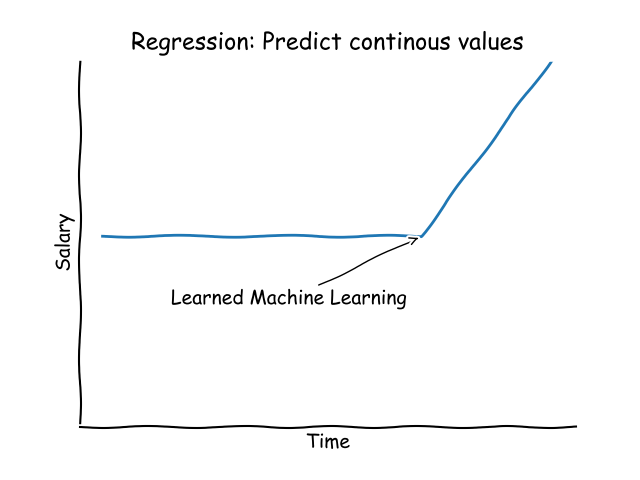We can help understand data by building mathematical models, this is key to machine learning.
One of such models is linear regression, in which we fit a line to (x,y) data.

There are many modules for Machine Learning in Python, but scikit-learn is a popular one.

Related course:

## Linear Regression

You may want to predict continous values. That is a regression problem. Linear Regression is one of the methods to solve that.

All kinds of values are continous: temperature, salary, numbers and many more. Regression is not always linear, as shown in the image below:But there are many data points on which linear regression can be applied. Like tay fare, where hours driven is directly correlated with the price to pay.

### Modules

Install the required modules;